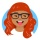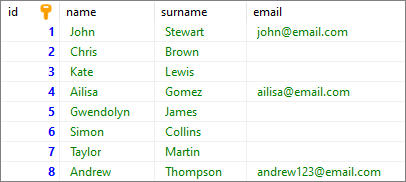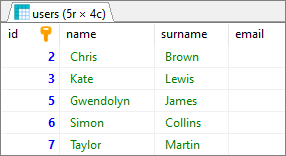Home Communities
IT Knowledge
Inspiration
Languages
EN

# MS SQL Server - find rows where column is empty string (blank)

0 points
Created by:Emelia
237

In this article, we would like to show you how to find rows where the column value is an empty string in MS SQL Server.

Quick solution:

``SELECT * FROM [table_name] WHERE [column_name] = '';``

If your column is NULL then the below query works:

``SELECT * FROM [table_name] WHERE [column_name] IS NULL;``

## Practical example

To show how to find rows where the column value is an empty string, we will use the following table:MS SQL Server - find rows with blank column - example data

Note:

At the end of this article you can find database preparation SQL queries.

### Example

In this example, we will find rows from `users` table with empty `email` column.

Query:

``````SELECT *
FROM [users]
WHERE [email] = '';``````

Result:MS SQL Server - find rows with blank column - result

## Database preparation

`create_tables.sql` file:

``````CREATE TABLE [users] (
[id] INT IDENTITY(1,1),
[name] VARCHAR(50) NOT NULL,
[surname] VARCHAR(50) NOT NULL,
[email] VARCHAR(50),
PRIMARY KEY ([id])
);``````

`insert_data.sql` file:

``````INSERT INTO [users]
( [name], [surname], [email])
VALUES
('John', 'Stewart', 'john@email.com'),
('Chris', 'Brown', ''),
('Kate', 'Lewis',''),
('Ailisa', 'Gomez', 'ailisa@email.com'),
('Gwendolyn', 'James', ''),
('Simon', 'Collins', ''),
('Taylor', 'Martin',''),
('Andrew', 'Thompson', 'andrew123@email.com');``````